# Solution assignment 03 Optimizing f(x)

### Assignment 3

Determine the extreme of the function: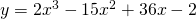and verify whether it is a maximum or a minimum by using the sign of the first derivative.

### Solution

In order to find the candidates for extremes we have to calculate the derivative and solve the following equation: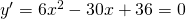thus: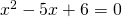and the solution of this quadratic equation is: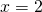or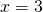Close tothe derivative has the following signs +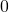- and thusis a maximum. Close tothe derivative has the following signs -+ and thus this point is a minimum.

0